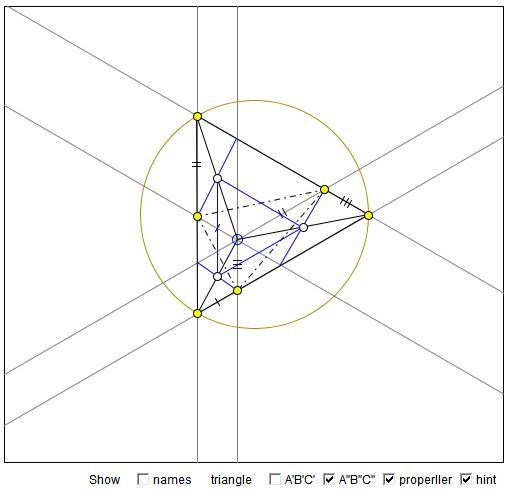# Pompeiu's TheoremWhat Is It? A Mathematical Droodle

### If you are reading this, your browser is not set to run Java applets. Try IE11 or Safari and declare the site https://www.cut-the-knot.org as trusted in the Java setup.What if applet does not run?

Explanation### Pompeiu's Theorem

The applet illustrates a theorem by D. Pompeiu (1873 - 1954)

For P not on the circumcircle of an equilateral ΔABC, there exists a triangle with side lengths equal to PA, PB, and PC.

(The triangle in question is known as Pompeiu's triangle.)For a proof, pass through P lines parallel to the sides to obtain points A', B', C' (or A'', B'', C'') on BC, AC, and AB, respectively. There are two triangles to consider: A'B'C' and A''B''C''.

Then, for example, quadrilateral A'CB'P is an isosceles trapezoid so that A'B' = PC. Similarly, B'C' = PA and C'A' = PB such that ΔA'B'C' is exactly Pompeiu's triangle. (For the triangle A''B''C'' the argument is similar. Naturally, the two triangles are congruent.)

For P on the circumcircle, Pompeiu's triangles degenerates into a straight line.

(There is another proof.)

Hubert Shutrick has observed that the triangles A'B'C' and A''B''C'' are obtained from each other by a rotation through 60°. This is because each of the three triangles PA'A'', PB'B'', PC'C'' is equilateral.

He found a relationship between angles in Pampeiu's triangle and and some angles in the base triangle. For example,

∠B'A'C' = ∠B'A'P + ∠C'A'P = ∠B'CP + ∠C'BP = ∠BPC - 60°.

This is because A'CB'P and A'BC'P are isosceles trapezoids which are cyclic polygons. Angles A'B'C' and A'C'B' are treated similarly.

Naturally, since triangles A'B'C' and A''B''C'' are congruent, the angles of the latter satisfy the same identities. Or, by direct argument,

∠B''A''C'' = ∠B''C''P + ∠C''A''P = ∠B''CP + ∠C''AP = ∠BPC - 60°.

Hubert also came up with an alternative proof for points P inside the triangle. The argument is very straightforward. Indeed, let PA be the longest among the three segments, PA, PB, PC. To prove the theorem suffice it to establish the inequality

PA < PB + PC.

However observed that PA is shorter than any of the sides of the triangle, BC in particular. Now, applying the triangle inequality to ΔPBC gives

PA < BC < PB + PC

and we are done.

As an aside, triangles A'B'C' and A''B''C'' mentioned previously form the configuration of the Asymmetric Propeller. The midpoints At, Bt, Ct of B''C', C''A', and A''B', respectively are the midpoints of PA, PB, and PC so that the equilateral Δ AtBtCt has sides parallel to those of ΔABC and is a homothetic image of the latter with center P and coefficient 1/2. This is so because, for example, the quadrilateral A'BC''P is a parallelogram, implying that the midpoint of A'C'' is also the midpoint of PB.

(There is a converse construction of finding an eqilateral triangle.)

### References

1. R. Honsberger, Mathematical Delights, MAA, 2004, 5-6
2. S. Savchev, T. Andreescu, Mathematical Miniatures, MAA, 2003, 89-90• Equilateral Triangles on Sides of a Quadrilateral
• Euler Line Cuts Off Equilateral Triangle
• Four Incircles in Equilateral Triangle
• Problem in Equilateral Triangle
• Problem in Equilateral Triangle II
• Sum of Squares in Equilateral Triangle
• Triangle Classification
• Isoperimetric Property of Equilateral Triangles
• Maximum Area Property of Equilateral Triangles
• Angle Trisectors on Circumcircle
• Equilateral Triangles On Sides of a Parallelogram
• Circle of Apollonius in Equilateral Triangle
•